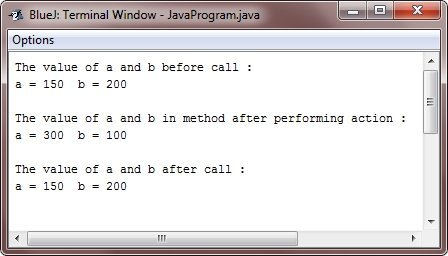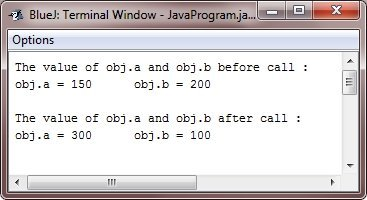# Java Call by Value and Call by Reference

## Java Parameter Passing

In general, there are the following two ways that a computer language can pass an argument to a subroutine :

1. call-by-value - The call-by-value approach copies the value of an argument into the formal parameter of the subroutine. Therefore, changes made to parameter of the function or subroutine have no effect on the argument.
2. call-by-reference - In call-by-reference approach, a reference to an argument (not the value of the argument) is passed to the parameter. Inside the subroutine, this reference is used to access the actual argument determined in the call. This means that changes made to the parameter will affect the argument used to call the subroutine.

## Java call by value

As you will see, Even though Java uses call-by-value to pass all the arguments, the precise effect differs between whether a primitive type or a reference type is passed.

Whenever you pass primitive type to a method, it is passed by value. Thus, a copy of the argument is made, and what happens to parameter that receives the argument has no effect outside the method. For example, consider the following example program :

## Java Call by Value Example

Here is an example program, helps you to understand, how to use call by value method to pass parameter to the method in Java:

```/* Java Program Example - Java Argument Passing
*  Primitive types are passed by value        */

class Test
{
void meth(int i, int j)
{
i = i * 2;
j = j / 2;

System.out.println("\nThe value of a and b in method after performing action :");
System.out.println("a = " + i + "\t b = " + j);
}
}

public class JavaProgram
{
public static void main(String args[])
{

Test obj = new Test();

int a = 150, b = 200;

System.out.println("The value of a and b before call :");
System.out.println("a = " + a + "\t b = " + b);

obj.meth(a, b);

System.out.println("\nThe value of a and b after call : ");
System.out.println("a = " + a + "\t b = " + b);

}
}```

When the above Java program is compile and executed, it will produce the following output:As you can see, the operations that occur in the method named meth() have no effect on the values of a and b used in the call, and their values here did not change to 300 and 100.

## Java Call by Reference

When you pass an object to a method, then the situation changes dramatically, because objects are passed by what is effectively call-by-reference.

Always keep in mind that when you create a variable of a class type, you are only creating a reference to an object. Therefore, when you pass this reference to a method, the parameter that receives it will refer to the same object as that referred to by the argument. This actually means that objects act as if they are passed to the methods by the use of call-by-reference. Changes to the object within the method do affect the object used as an argument. For example, consider the following program :

## Java Call by Reference Example

Below is an example program given, uses call by reference method, to pass argument/parameter to the method in Java:

```/* Java Program Example - Java Argument Passing
*  Objects are passed through their reference   */

class Test
{
int a, b;

Test(int i, int j)
{
a = i;
b = j;
}

/* pass an object */
void meth(Test o)
{
o.a = o.a * 2;
o.b = o.b / 2;
}
}

public class JavaProgram
{
public static void main(String args[])
{

Test obj = new Test(150, 200);

int a = 150, b = 200;

System.out.println("The value of obj.a and obj.b before call :");
System.out.println("obj.a = " + obj.a + "\t obj.b = " + obj.b);

obj.meth(obj);

System.out.println("\nThe value of obj.a and obj.b after call : ");
System.out.println("obj.a = " + obj.a + "\t obj.b = " + obj.b);

}
}```

When the above Java program is compile and executed, it will produce the following output:As you can see, in this case, the actions inside the meth() have affected the object used as an argument.

Tools
Calculator